Next: Uses of entropy Up: Statistical thermodynamics Previous: Entropy

## Properties of entropy

Entropy, as we have defined it, has some dependence on the resolution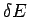to which the energy of macrostates is measured. Recall that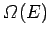is the number of accessible microstates with energy in the rangeto. Suppose that we choose a new resolution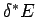and define a new density of states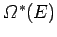which is the number of states with energy in the rangeto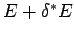. It can easily be seen that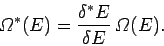(227)

It follows that the new entropy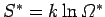is related to the previous entropy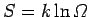via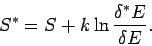(228)

Now, our usual estimate that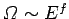gives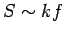, where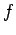is the number of degrees of freedom. It follows that even ifwere to differ fromby of order(i.e., twenty four orders of magnitude), which is virtually inconceivable, the second term on the right-hand side of the above equation is still only of order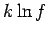, which is utterly negligible compared to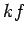. It follows that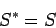(229)

to an excellent approximation, so our definition of entropy is completely insensitive to the resolution to which we measure energy (or any other macroscopic parameter).

Note that, like the temperature, the entropy of a macrostate is only well-defined if the macrostate is in equilibrium. The crucial point is that it only makes sense to talk about the number of accessible states if the systems in the ensemble are given sufficient time to thoroughly explore all of the possible microstates consistent with the known macroscopic constraints. In other words, we can only be sure that a given microstate is inaccessible when the systems in the ensemble have had ample opportunity to move into it, and yet have not done so. Note that for an equilibrium state, the entropy is just as well-defined as more familiar quantities such as the temperature and the mean pressure.

Consider, again, two systemsand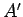which are in thermal contact but can do no work on one another (see Sect. 5.2). Letand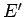be the energies of the two systems, andandthe respective densities of states. Furthermore, let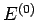be the conserved energy of the system as a whole and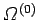the corresponding density of states. We have from Eq. (158) that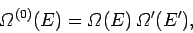(230)

where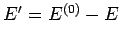. In other words, the number of states accessible to the whole system is the product of the numbers of states accessible to each subsystem, since every microstate ofcan be combined with every microstate ofto form a distinct microstate of the whole system. We know, from Sect. 5.2, that in equilibrium the mean energy oftakes the value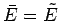for which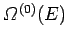is maximum, and the temperatures ofandare equal. The distribution ofaround the mean value is of order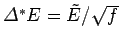, whereis the number of degrees of freedom. It follows that the total number of accessible microstates is approximately the number of states which lie within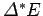of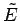. Thus,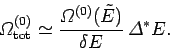(231)

The entropy of the whole system is given by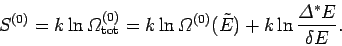(232)

According to our usual estimate,, the first term on the right-hand side is of orderwhereas the second term is of order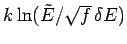. Any reasonable choice for the energy subdivisionshould be greater than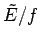, otherwise there would be less than one microstate per subdivision. It follows that the second term is less than or of order, which is utterly negligible compared to. Thus,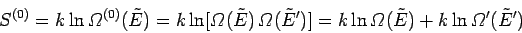(233)

to an excellent approximation, giving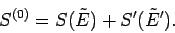(234)

It can be seen that the probability distribution foris so strongly peaked around its maximum value that, for the purpose of calculating the entropy, the total number of states is equal to the maximum number of states [i.e.,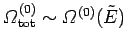]. One consequence of this is that the entropy has the simple additive property shown in Eq. (234). Thus, the total entropy of two thermally interacting systems in equilibrium is the sum of the entropies of each system in isolation.Next: Uses of entropy Up: Statistical thermodynamics Previous: Entropy
Richard Fitzpatrick 2006-02-02